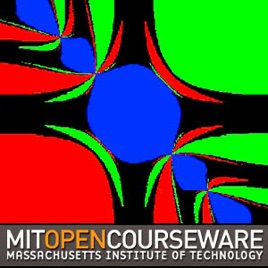6エピソード

The collection includes six supplementary lectures demonstrating how to use MATLAB's command prompt, plot graphs, use files and functions, and debug code.

Album art image by Yossi Farjoun.# Introduction to MATLAB Programming MIT

• 教育

The collection includes six supplementary lectures demonstrating how to use MATLAB's command prompt, plot graphs, use files and functions, and debug code.

Album art image by Yossi Farjoun.

• video
Lecture 1: Using MATLAB for the First Time

## Lecture 1: Using MATLAB for the First Time

This video lecture introduces the MATLAB interface, how to carry out basic mathematical operations involving both numbers and variables, and how to use the help files to learn more about MATLAB's built-in functions.

• 5分
• video
Lecture 2: The Command Prompt

## Lecture 2: The Command Prompt

This video lecture continues to explore MATLAB's command prompt and function library, as well as provides an introduction to plotting and creating for and while loops.

• 7分
• video
Lecture 3: Using Files

## Lecture 3: Using Files

This video lecture demonstrates how to create code in the editor and save it in a file to be used as a function or script. Examples include a script for the Fibonacci sequence and a function that converts Fahrenheit to Celsius.

• 10分
• video
Lecture 4: Plotting

## Lecture 4: Plotting

This video lecture demonstrates how to manipulate the style, axes, and position of plots in MATLAB and how to create multiple subplots.

• 7分
• video
Lecture 5: Scripts and Functions

## Lecture 5: Scripts and Functions

This video lecture demonstrates the concept of scope by example of a recursive function that produces the Fibonacci sequence.

• 9分
• video
Lecture 6: Debugging

## Lecture 6: Debugging

This video demonstrates how to use MATLAB's built-in debugger to track variables, values, and function returns.

• 10分